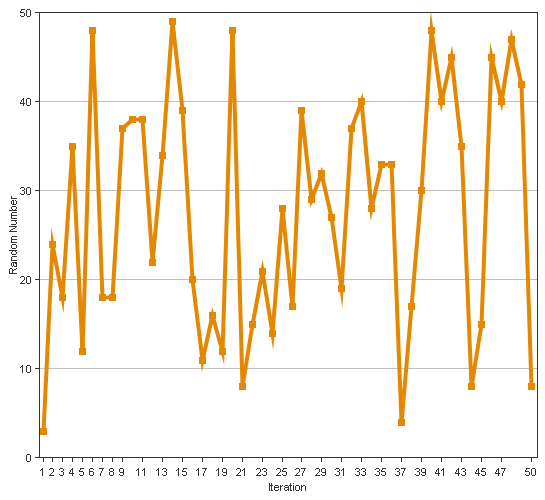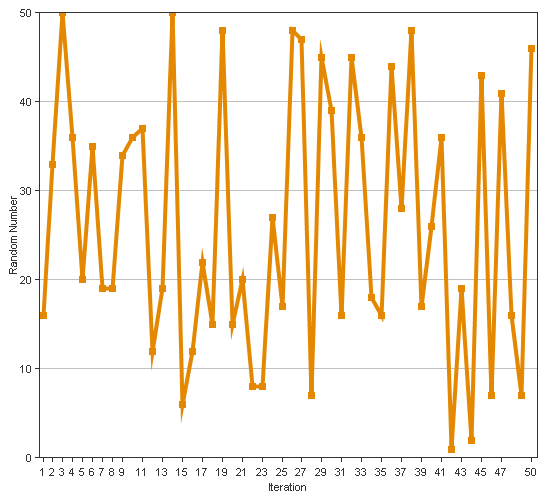# Comparing ColdFusion Number Randomization Algorithms

By on
Tags:

The other week, I posted about how I didn't feel like ColdFusion always did a great job of random number generation. When using ColdFusion's RandRange() method, I just pass in the two required integers. After posting this, Dustin told me that ColdFusion MX7 introduced a third argument for the RandRange() method, which was the algorithm by which the random numbers were generated. By default, ColdFusion uses the CFMX_COMPAT algorithm. Apparently (and this is stated directly in the documentation), the alternate algorithm, SHA1PRNG, will do a much better job of randomizing numbers.

To explore these two algorithms, I first wanted to start out graphing them, to see if I could see any obvious trends. In the following example, I am looping over the two algorithms and then using ColdFusion's CFChart tag to charge 50 random numbers between the values 1 and 50.

``````<!---
Loop over the two algorithms, the default
CFMX_COMPAT and then the SHA1PRNG. We are
going to chart some random numbers to see
what they look like.
--->
<cfloop
index="strAlgorithm"
list="CFMX_COMPAT,SHA1PRNG"
delimiters=",">

<!---
Create a line graph of this randomly
selected numbers.
--->
<cfchart
format="png"
chartheight="500"
chartwidth="545"
labelformat="number"
xaxistitle="Iteration"
yaxistitle="Random Number">

<cfchartseries type="line">

<!---
Create each data item by randomly generating
a number using one of the algorithms.
--->
<cfloop
index="intI"
from="1"
to="50"
step="1">

<cfchartdata
item="#intI#"
value="#RandRange( 1, 50, strAlgorithm )#"
/>

</cfloop>

</cfchartseries>

</cfchart>

</cfloop>
``````

From the above code, we get the following graphs:

Algorithm: CFMX_COMPAT (ColdFusion's Default)Algorithm: SHA1PRNG (Added in ColdFusion MX7)Now, I look at these two graphs, and frankly, they don't mean anything to me. I don't see trends, and even if I do see some trends, I don't understand the significance. Both of these graphics look like a nice randomized set of numbers.

But more than that, this is not a useful test case for me. My issues with randomness rarely involve generating a ton of numbers right in a row; my scenarios usually involve manually refreshing a page to see if something is rotating "properly" (think advertisements or header images). In that case, there is a big delay between random number generation (compared the delay between CFLoop iterations). In my next experiment, I am using a META tag refresh to put a uniform delay between my page refreshes as this will most closely mimic me sitting there and hitting the browser's refresh button:

``````<!--- Param the list of random numbers. --->
<cfparam
name="URL.numbers"
type="string"
default=""
/>

<!---
Create a random number using one of the
two algorithms, CFMX_COMPAT or SHA1PRNG.
--->
<cfset intNumber = RandRange( 1, 10, "SHA1PRNG" ) />

<!--- Add it to the list of numbers. --->
<cfset URL.numbers = ListAppend( URL.numbers, intNumber ) />

<!---
Check to see if we have generated enough numbers.
We want to generate 20. If have less than 20, let
provide the refresh link. If we have 20, just output
the numbers.
--->
<cfif (ListLen( URL.numbers ) LT 20)>

<!---
Provide meta-drive refresh. This is to ensure
that the timing of the refresh is similar for
each page refresh.
--->
<meta
http-equiv="refresh"
content=".5; url=#CGI.script_name#?numbers=#URL.numbers#"
/>

<cfelse>

<!--- We have all the numbers, so output them. --->
#URL.numbers#

</cfif>
``````

I ran the above code three times for each algorithm and here are the number lists that were generated:

Algorithm: CFMX_COMPAT (ColdFusion's Default)

• 9,7,7,7,7,7,7,6,6,6,6,9,9,8,9,8,8,8,8,8

• 3,6,6,6,5,6,6,5,5,4,4,5,2,3,2,2,2,2,2,2

• 5,8,8,7,7,6,7,7,6,6,9,10,9,9,8,8,9,8,8,8

Algorithm: SHA1PRNG (Added in ColdFusion MX7)

• 6,8,9,6,4,5,5,9,8,4,10,5,7,4,4,8,10,2,6,1

• 6,6,8,9,8,1,8,9,9,3,7,3,3,5,6,7,7,3,4,5

• 4,8,2,8,4,1,4,4,3,3,9,10,2,10,6,6,4,4,5,2

Just looking at these numbers, I can clearly see grouping in the CFMX_COMPAT algorithm. There is some grouping in the SHA1PRNG algorithm, but to a much much lesser degree. I don't know how the timing of the random number generation affects things, but it seems to have some sort of a link to the seemingly effective nature of the outcome. Now, I say "seemingly" because, remember, I am really more concerned about an even distribution of numbers and less so about the actual randomization of the numbers. Randomization or not, the SHA1PRNG seems to have a better distribution of numbers.

Want to use code from this post? Check out the license.Here's the mathematical proof for the variance (http://en.wikipedia.org/wiki/Variance) of the above results.

CFMX_COMPAT - variance - standard dev
test 1 - 1.05 - 1.0246
test 2 - 2.69 - 1.6401
test 3 - 1.5275 - 1.2359

SHA1PRNG - variance - standard dev
test 1 - 6.1475 - 2.4794
test 2 - 5.4275 - 2.3296
test 3 - 7.1475 - 2.6734

As the numbers get larger, the more variance the test set has present. A standard deviation of 2 means you should be hitting ~95% of your population, where 1 is only ~68%. The proof is in the pudding so to speak (sorry for the math pun).

I used this UDF to calculate the variance: http://www.cflib.org/udf.cfm?ID=256@Dustin,

It's been a million years since I took a statistics class (and didn't do so well in it). From what it looks like, a bigger standard deviation is a Good thing since, if you think about the Bell Curve, you are covering more ground (as you say, I think). Thanks for doing the testing.Yeah, it has been a long time for me as well. In fact I forgot about normal vs. random distributions when talking about standard deviation. The confidence levels stated (~95% and ~64%) are for a normal distribution, which we aren't dealing with. For a random distribution it is ~75% for 2 stddevs and ~50% for 1.41 stddevs.

I'm beginning to remember why I didn't particularity care for the class.Yeah, if I never hear about a z-test or t-square test (or something like that) again, I will quite content. My brain just doesn't seem to like that sort of thing.Thanks Ben,

I was complaining about the default behavior of randrange to a colleague only 2 days ago when observing the behavior of an online competition application we were running.

Little did I know that this behavior could be changed!

All goes to show I should RTFM!